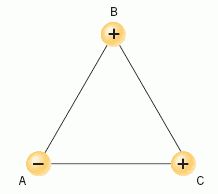# Net Force of Charges on an Equilateral Triangle

## Homework Statement

Three point charges have equal magnitudes, two being positive and one negative.
These charges are fixed to the corners of an equilateral triangle.
The magnitude of each of the charges is 2.9 µC.
The lengths of the sides of the triangle are .02m
Calculate the magnitude of the net force that each charge experiences.## Homework Equations

The only equation my professor gave me was:
F = kQq / r2

## The Attempt at a Solution

I used F = kQq / r2 to get F. I got 189 N, which I think should be the magnitudes of net force at points B and C. But I don't know what to do now to get point A. I tried using other formulas I found on the web, but they keep giving me the wrong answer. Help please?

Simon Bridge
Homework Helper
A each corner, the charges will experience a force due to both the other two.
Recall that force is a vector.

So what am I supposed to do? Fnet = F1 + F2, but where do the vectors come in? I'm not sure which ones should be negative or positive... How do I tell?

Simon Bridge
Homework Helper
You know a rule for which way two charges pull or push each other.
"Opposite charges _______________." (complete the sentence)

It's direction that's important - do you know how to add vectors?

Opposite charges attract... I forgot how to add vectors... I saw one formula that multiplied by sin or cos. I assume I use sin if it's got a y component, cos if x. I'm sorry, my professor hasn't taught me a thing about this stuff, and I can't afford the textbook...

Simon Bridge